# How to calculate lookup column in SharePoint list

SharePoint Calculated column is very powerful when creating out-of-the-box solutions. You can use it to calculate values from other columns. SharePoint Calculated Column supports almost column types, but does not support lookup column. So if you want to calculate value of lookup column, you need to try some methods, such as SharePoint Designer workflow.

This example demonstrate how to utilize the workflow.

This is a Product list which is used to record the product details, including Product Name, Unit, Number per Unit and Unit Price.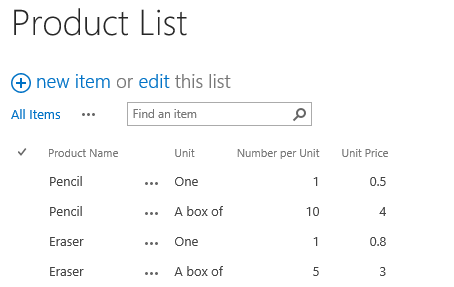And there is a Shopping list which is used to record purchasing information. And the Product field is lookup from the Product list. Unit, Number per Unit and Unit Price columns are additional fields of lookup column.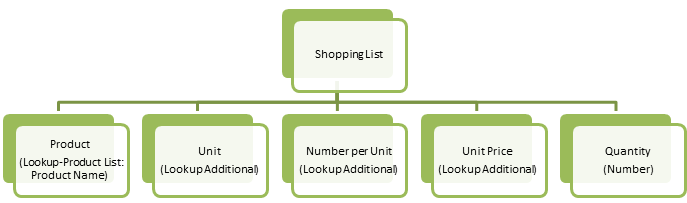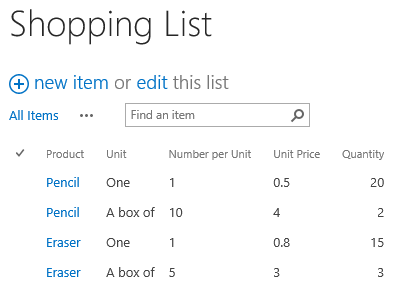Here I want to calculate the Total Price，Total Number of Product, and generate Shopping Details using the Unit and Number per Unit columns.

One Solution is to create a Number column called Unit Price(Number), and assign the value of Unit Price (which is a lookup column) to this column utilizing a workflow. Then create a calculated column and type this formula: =[Unit Price(Number)]*[Quantity].

Following steps demonstrate how to do this:

a Create a Number column and name it Unit Price(Number).

b. Open the SharePoint Designer, and configure the Update List Items workflow as following screenshot.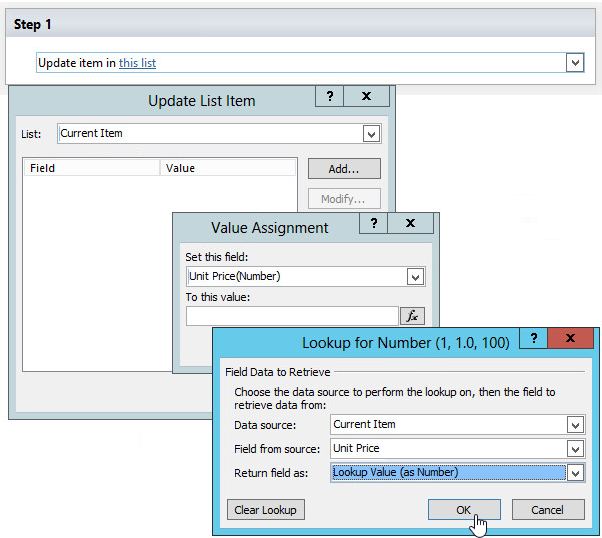c. Create a SharePoint calculated column, and design the formula as ‘=[Unit Price(Number)]*[Quantity]’.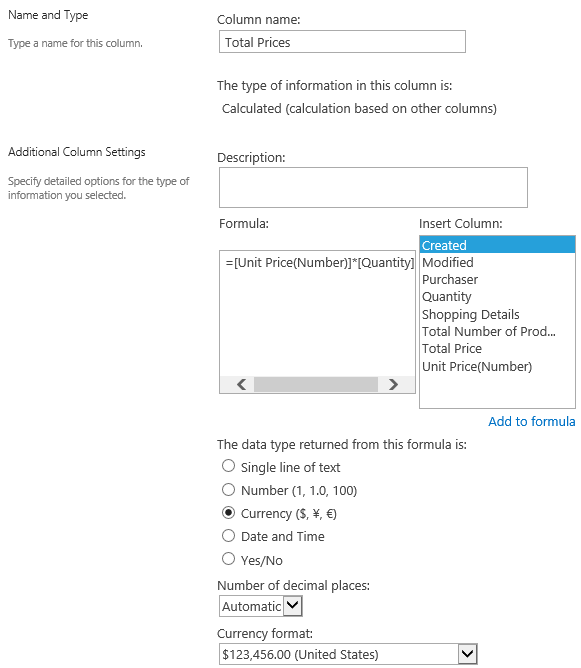Repeat above steps, we can calculate Total Number of Product, but I need to create some Number columns and workflows, and they are not necessary in the list.

Now let’s try to solve this problem using SharePoint Calculated Boost column.

First of all, install and deploy Calculated Boost on your SharePoint server. Then create a Calculated Boost column named Total Price and design the formula as ‘=[Unit Price]*[Quantity]’. Note that the Price Unit column is the lookup column. And set the output type of this column as Currency.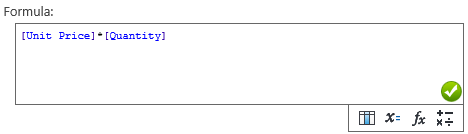And then create another Calculated Boost column and named Total Number of Product, and design the formula as following screenshot. Set the output type as Number.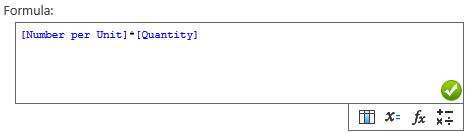Even better, you can create a formula in Calculated Boost column to manipulate text. Here I create the following formula to generate details.

And set the output type as Single Line of Text.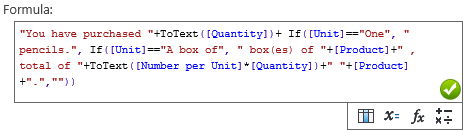And then, you can see the results as followings.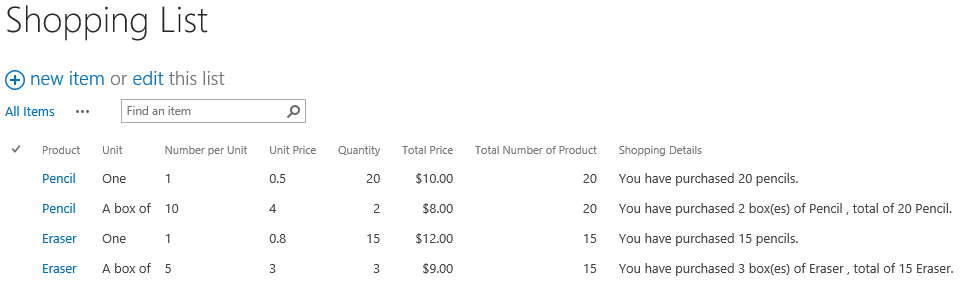### 3 Responses

1.Tyler Schwerdt at |

So when I try to create the workflow I don’t see the correct option for the “Field from Source” pull down. What could I be missing?

2.elin li at |

when I’m using sharepoint designer lookup for number, it can’t find the additional fields from my sharepoint list lookup. anyone know how to solve this?

3.Jonie Pangelinan at |

This is a great blog. and i want to visit this every day of the week ,